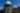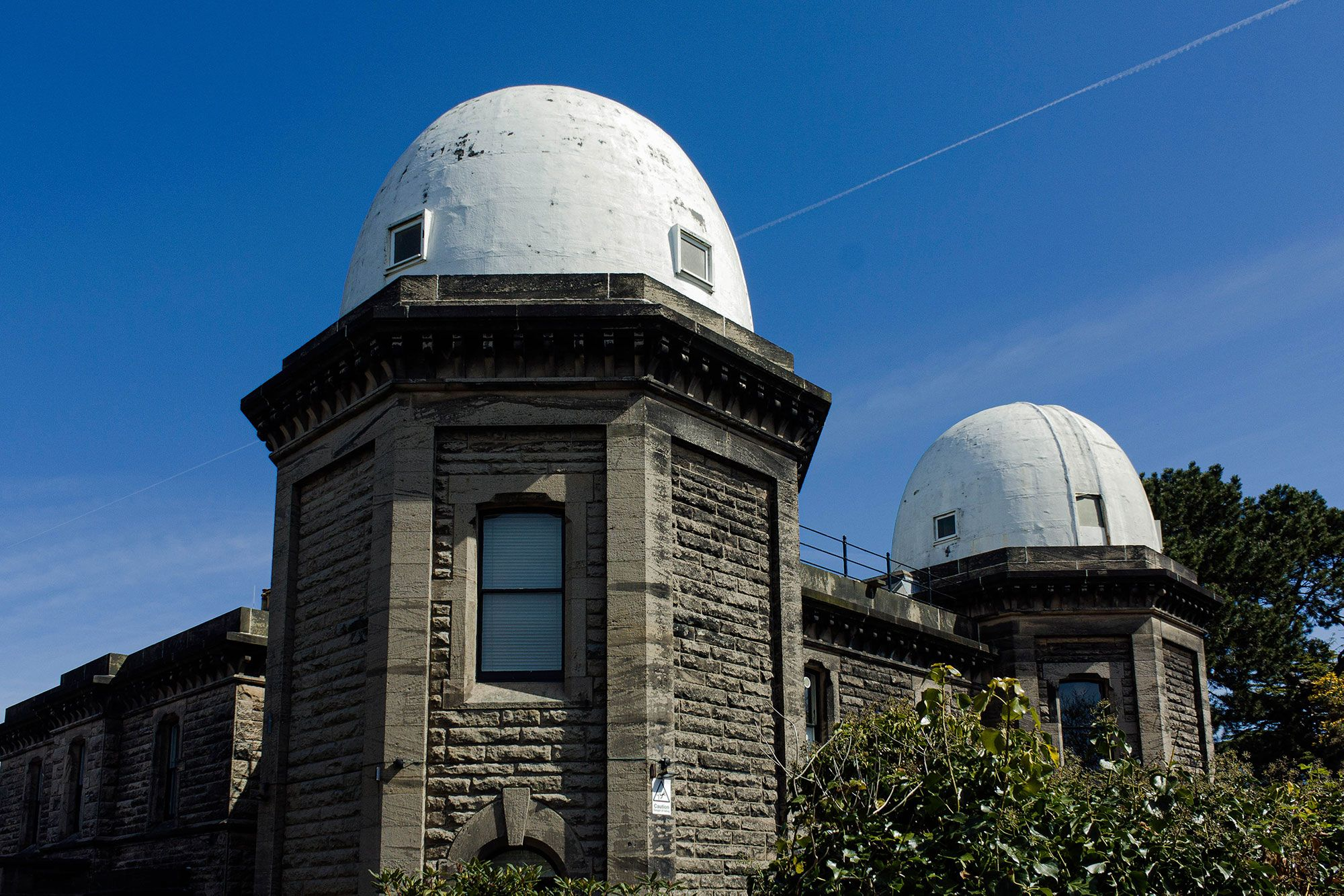## ((((((((((((((((((((((((((((((((((((((((((((((((((((((((((((((((((((((((((((((((((((((((((((((((((((((((((((((((((((((((((((((((((((((((((((((((((((((((((((((((((((((((((((((((((((((((((((((((((((((((The Project))))))))))))))))))))))))))))))))))))))))))))))))))))))))))))))))))))))))))))))))))))))))))))))))))))))))))))))))))))))))))))))))))))))))))))))))))))))))))))))))))))))))))))))))))))))))))))))))))))))))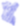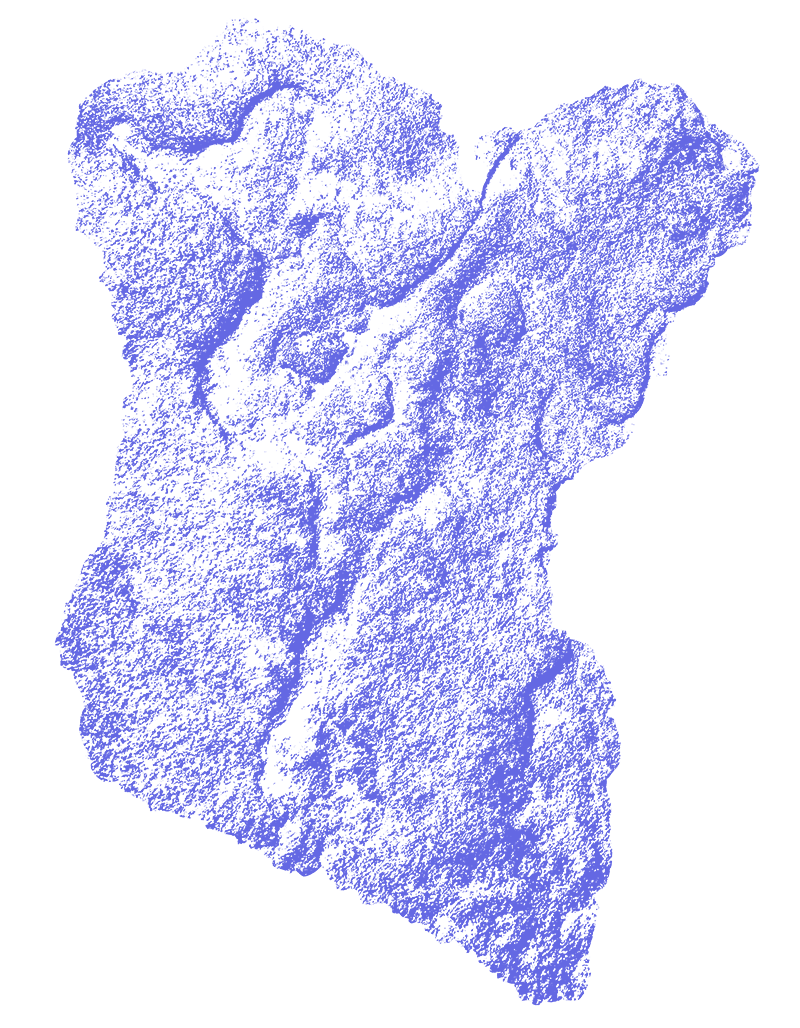Bidston Observatory Artistic Research Centre (BOARC) is a self-organising, communal study site. It is a not-for-profit project that focuses on providing individual users and groups with a low cost, temporary place to work and stay together; to develop projects that require time and space in a flexible, non-pressured environment.

BOARC is a site for research and experimentation, which is primarily directed towards cultural and artistic practice, and supporting the development of communities. It is a space where those invested in culture, social justice and welfare, from a range of fields can come together to share, test and extend ideas and mutually support each other. BOARC is open to a range of academic and nonacademic discourses, bodywork, collective choreography, and chat - it is a space where discussion can flow from kitchen to sound recording studio, and ideas can bounce between the domes and basements.

Main kitchen and workspace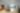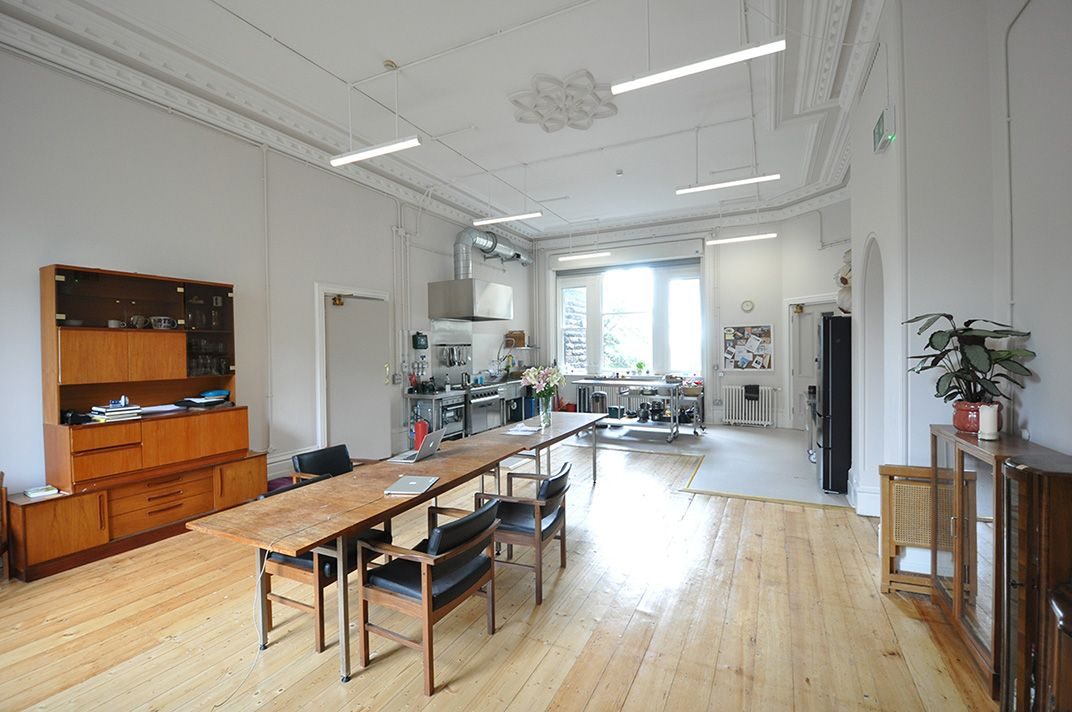There is no formal application to use the resource and BOARC does not look to lay claim to work produced here or put focus on presentation. In this sense, it is not a typical artists' residency site. Stayers and day users commit to their projects, and practice daily communal maintenance of the domestic and work spaces.

Find out more about our events here

BOARC also hosts seminars, events and workshops throughout the year.

Basement workspace, below main kitchen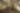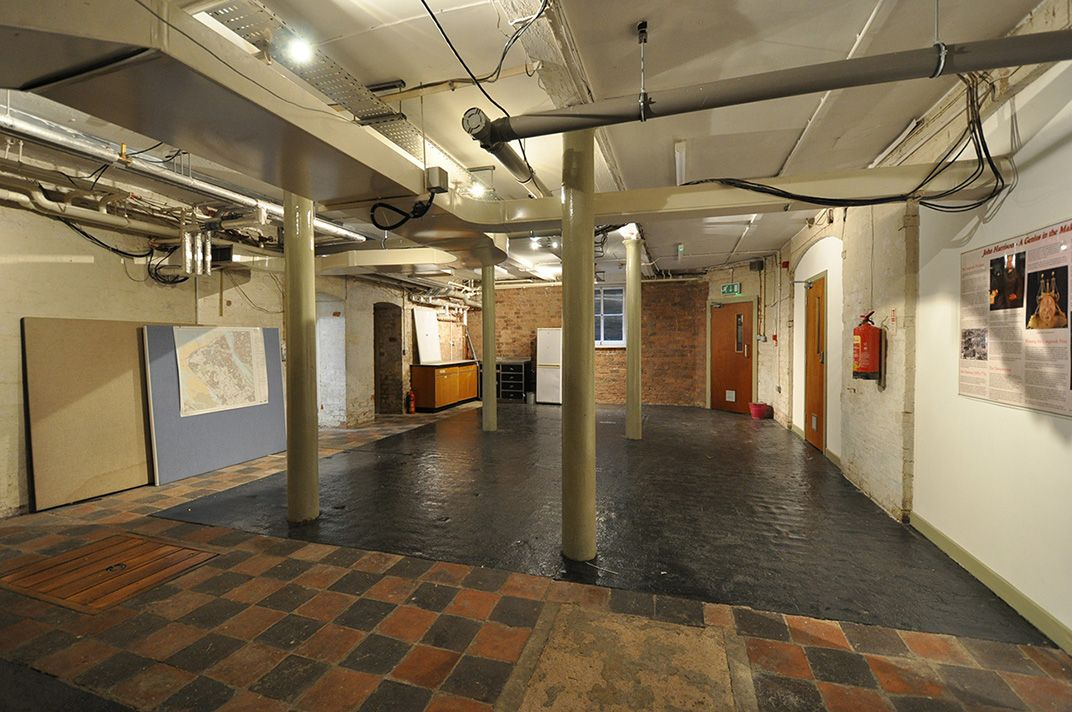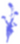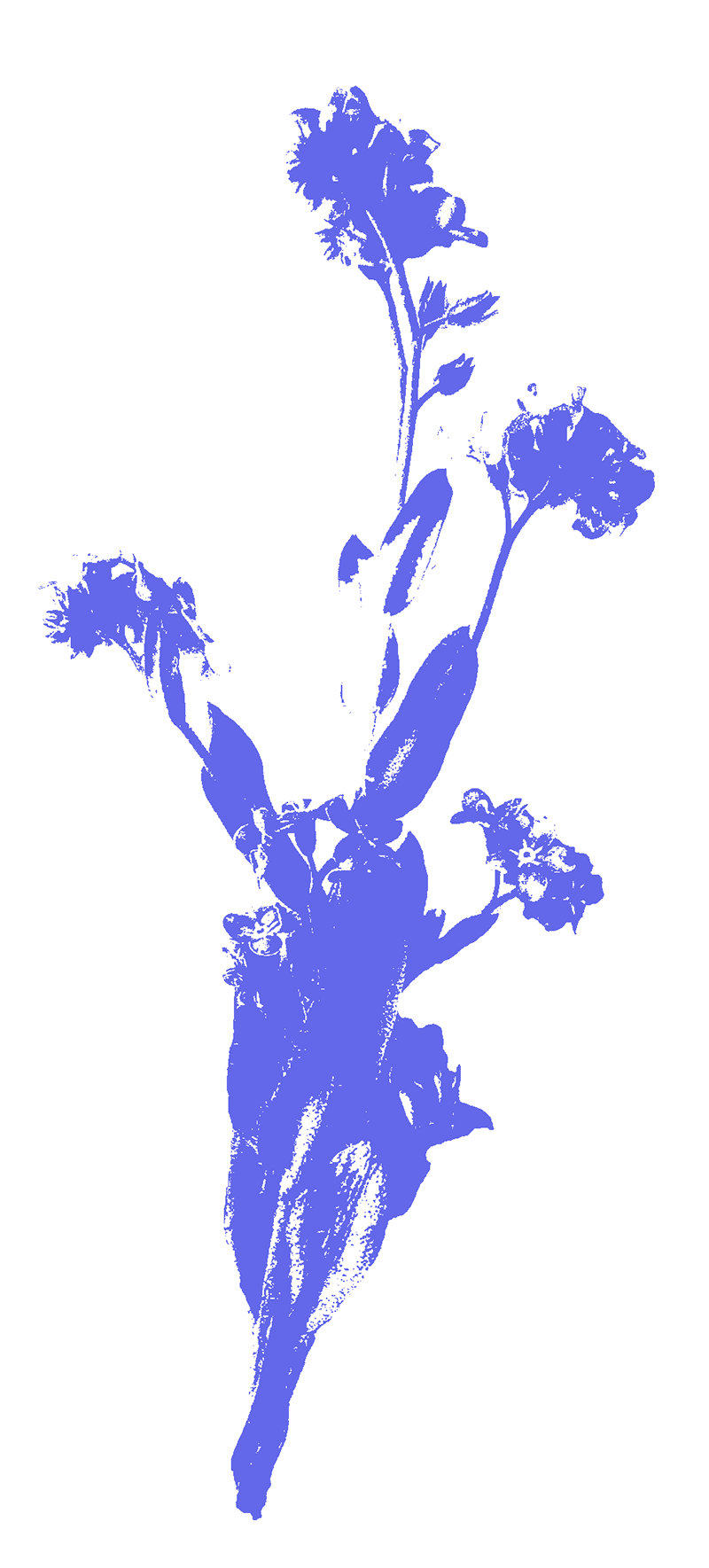## ((((((((((((((((((((((((((((((((((((((((((((((((((((((((((((((((((((((((((((((((((((((((((((((((((((((((((((((((((((((((((((((((((((((((((((((((((((((((((((((((((((((((((((((((((((((((((((((((((((((((Values and Accountability))))))))))))))))))))))))))))))))))))))))))))))))))))))))))))))))))))))))))))))))))))))))))))))))))))))))))))))))))))))))))))))))))))))))))))))))))))))))))))))))))))))))))))))))))))))))))))))))))))))))

BOARC uses the following principles as guides for working and staying together at the site:

Efflorescence in the basement. Crystalline salt deposits forming in the basement work spaces as they dried out during renovations.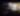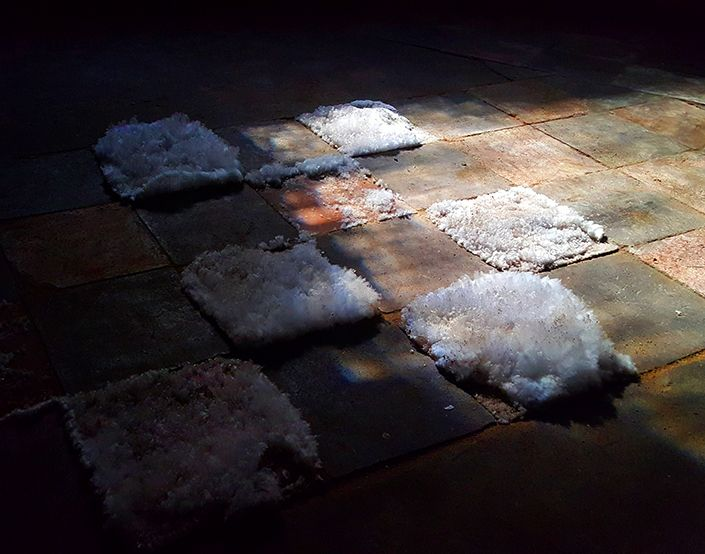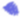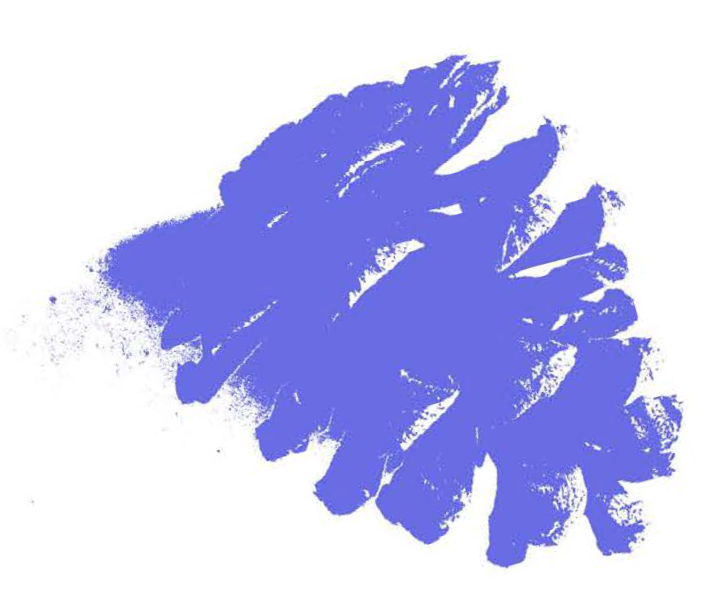Principles for being together at BOARC have been established over the course of getting the project up and running, and through discussions with the project initiators and multiple collaborators. They emerge from intersectional feminist values and practices.

Stayers and day users should read these principles if they intend to use the resource. It is not essenatial to agree with them exactly as they are written. However, they offer a feel for the values and practices enacted in and envisoned for the space.

In using BOARC, you are agreeing to practice the accountability agreement. Please read the accountability agreement thoroughly.

This project is aiming to experiment with different ways of doing things, and we invite your thoughts! We’re constantly developing our processes and we’d love your input as we keep creating them. If you have anything to say about the BOARC Accountability Agreement, or any aspect of the BOARC project, please let us know.

About the project’s organisation

Bidston Observatory is privately owned, bought in 2016 by two of the current directors specifically for this project. The purchase and renovations were funded by the sale of hereditary land in the West Country for commercial and residential development. The property is leased to BOARC for what is known as a ‘peppercorn rent’ – BOARC pays a peppercorn (literally) each year to the owners. The owners maintain the building, often themselves. This structure makes it possible to keep stayer and user costs low. No property costs are passed on to stayers and the directors and owners have no intention to profit from users of the building.

Bidston Observatory Artistic Research Centre was incorporated as a PLC in 2019, and is run as a not-for-profit. There are currently five directors who meet once a quarter to guide the project. The directorship is unpaid. You’ll probably meet one or two directors during your stay as three work every week onsite as stewards. There is a small team of stewards who support the running of the project. They answer mails, plan events, help clean and everything in between. Some stewards are volunteers and some are paid.

BOARC is an experiment in itself, which takes energy, good humour, self-reflection and lots of patience to run, and we thank you for your participation!

## ((((((((((((((((((((((((((((((((((((((((((((((((((((((((((((((((((((((((((((((((((((((((((((((((((((((((((((((((((((((((((((((((((((((((((((((((((((((((((((((((((((((((((((((((((((((((((((((((((((((((Opportunities))))))))))))))))))))))))))))))))))))))))))))))))))))))))))))))))))))))))))))))))))))))))))))))))))))))))))))))))))))))))))))))))))))))))))))))))))))))))))))))))))))))))))))))))))))))))))))))))))))))))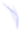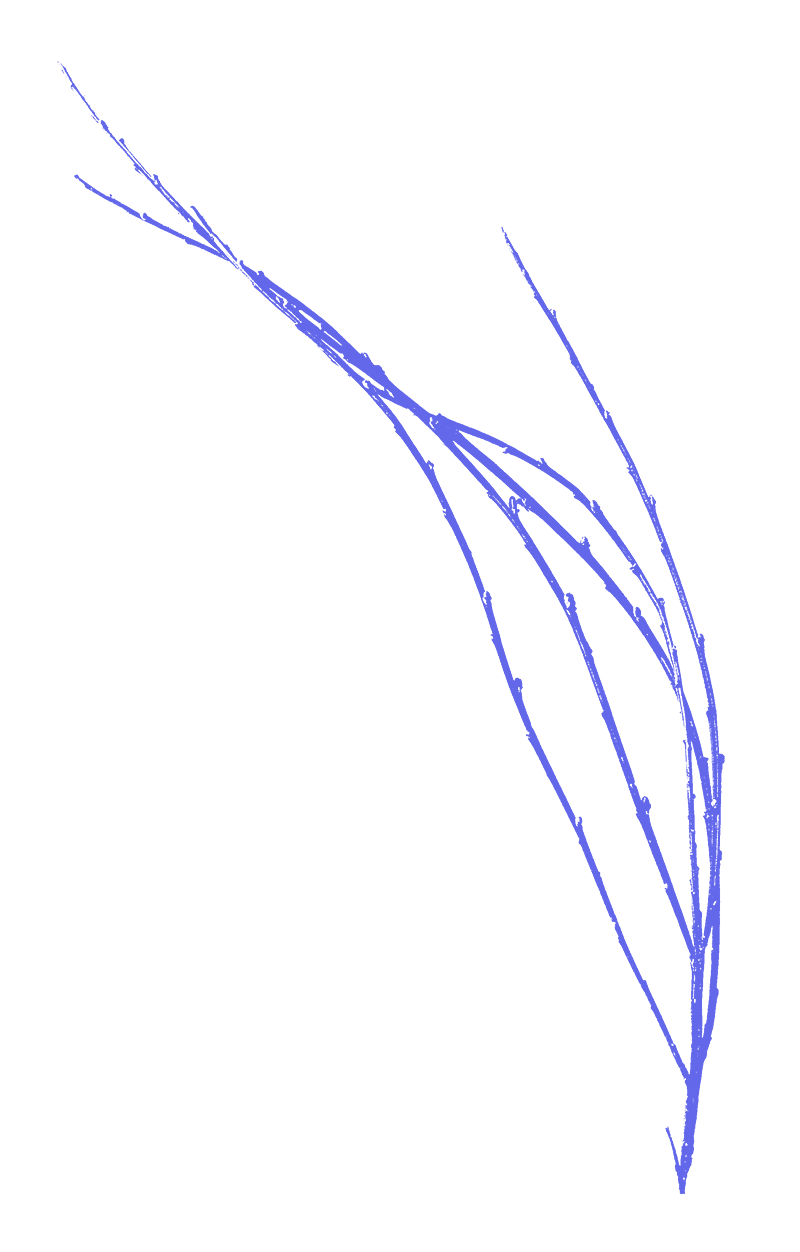+
+
+
+
+